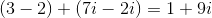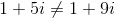## Example Questions

### Example Question #4 : Complex Numbers

Suppose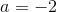and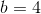Evaluate the following expression: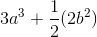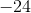Explanation:

Substituting forand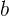, we have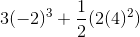This simplifies to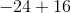which equals### Example Question #5 : Complex Numbers

What is the solution of the following equation?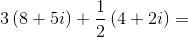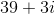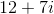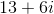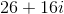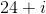Explanation:

A complex number is a combination of a real and imaginary number. To add complex numbers, add each element separately.

First, distribute: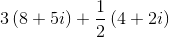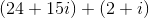Then, group the real and imaginary components: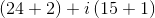Solve to get:### Example Question #6 : Complex Numbers

What is the sum ofandgiven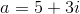and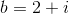?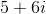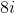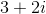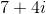Explanation:

A complex number is a combination of a real and imaginary number. To add complex numbers, add each element separately.

In equationis the real component andis the imaginary component (designated by).

In equationis the real component andis the imaginary component.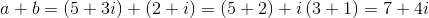### Example Question #7 : Complex Numbers

Complex numbers take the form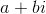, where a is the real term in the complex number and bi is the nonreal (imaginary) term in the complex number.

Simplify: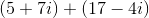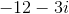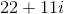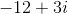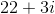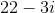Explanation:

When adding or subtracting complex numbers, the real terms are additive/subtractive, and so are the nonreal terms.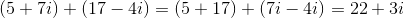### Example Question #8 : Complex Numbers

Complex numbers take the form, where a is the real term in the complex number and bi is the nonreal (imaginary) term in the complex number.

Can you add the following two numbers: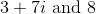? If so, what is their sum?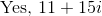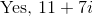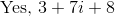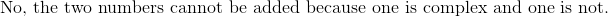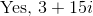Explanation:

Complex numbers take the form a + bi, where a is the real term in the complex number and bi is the nonreal (imaginary) term in the complex number. Taking this, we can see that for the real number 8, we can rewrite the number as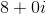, where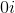represents the (zero-sum) non-real portion of the complex number.

Thus, any real number can be added to any complex number simply by considering the nonreal portion of the number to be.

### Example Question #1 : How To Add Complex Numbers

Complex numbers take the form, whereis the real term in the complex number and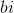is the nonreal (imaginary) term in the complex number.

Which of the following is incorrect?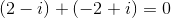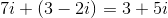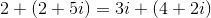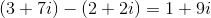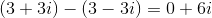Explanation:

Complex numbers take the form, whereis the real term in the complex number andis the nonreal (imaginary) term in the complex number.

Thus, to balance the equation, add like terms on the left side.